Before you go through this article, make sure that you have gone through the previous article on Ripple Carry Adder.

• Each full adder has to wait for its carry-in from its previous stage full adder.
• Thus, nth full adder has to wait until all (n-1) full adders have completed their operations.
• This causes a delay and makes ripple carry adder extremely slow.
• The situation becomes worst when the value of n becomes very large.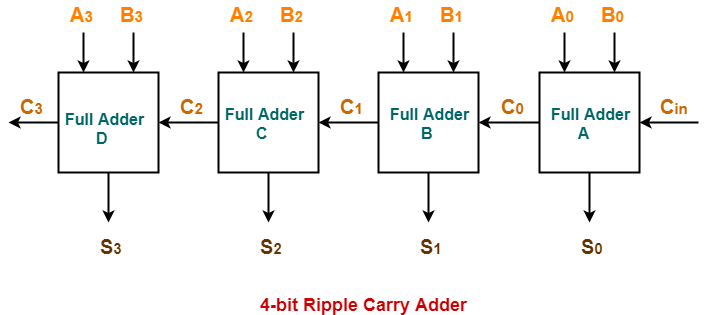• It generates the carry-in of each full adder simultaneously without causing any delay.
• The time complexity of carry look ahead adder = Θ (logn).

## Logic Diagram-

The logic diagram for carry look ahead adder is as shown below-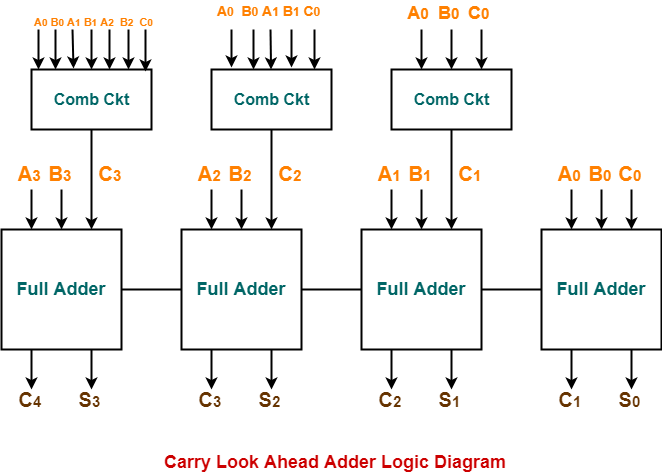The working of carry look ahead adder is based on the principle-The carry-in of any stage full adder is independent of the carry bits generated during intermediate stages.

The carry-in of any stage full adder depends only on the following two parameters-

• Bits being added in the previous stages
• Carry-in provided in the beginning

Now,

• The above two parameters are always known from the beginning.
• So, the carry-in of any stage full adder can be evaluated at any instant of time.
• Thus, any full adder need not wait until its carry-in is generated by its previous stage full adder.

Consider two 4-bit binary numbers A3A2A1A0 and B3B2B1B0 are to be added.

Mathematically, the two numbers will be added as-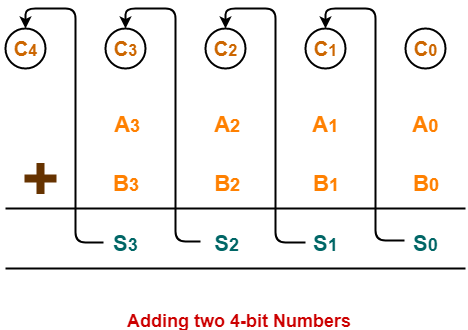From here, we have-

C1 = C0 (A0 ⊕ B0) + A0B0

C2 = C1 (A1 ⊕ B1) + A1B1

C3 = C2 (A2 ⊕ B2) + A2B2

C4 = C3 (A3 ⊕ B3) + A3B3

For simplicity, Let-

• Gi = AiBi where G is called carry generator
• Pi = Ai ⊕ Bi where P is called carry propagator

Then, re-writing the above equations, we have-

C1 = C0P0 + G………….. (1)

C2 = C1P1 + G1 ………….. (2)

C3 = C2P2 + G2 ………….. (3)

C4 = C3P3 + G3 ………….. (4)

Now,

• Clearly, C1, C2 and C3 are intermediate carry bits.
• So, let’s remove C1, C2 and C3 from RHS of every equation.
• Substituting (1) in (2), we get C2 in terms of C0.
• Then, substituting (2) in (3), we get C3 in terms of C0 and so on.

Finally, we have the following equations-

• C1 = C0P0 + G
• C2 = C0P0P1 + G0P1 + G1
• C3 = C0P0P1P2 + G0P1P2 + G1P2 + G2
• C4 =C0P0P1P2P3 + G0P1P2P3 + G1P2P3 + G2P3 + G3

These equations are important to remember.

These equations show that the carry-in of any stage full adder depends only on-

• Bits being added in the previous stages
• Carry bit which was provided in the beginning

### Trick To Memorize Above Equations-

As an example, let us consider the equation for generating carry bit C2.

There are three possible reasons for generation of C2 as depicted in the following picture-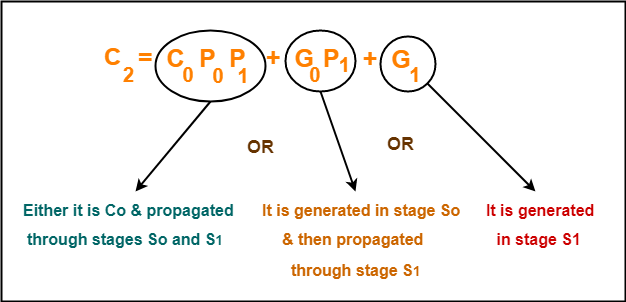In the similar manner, we can write other equations as well very easily.

## Implementation Of Carry Generator Circuits-

The above carry generator circuits are usually implemented as-

• Two level combinational circuits.
• Using AND and OR gates where gates are assumed to have any number of inputs.

### Implementation Of C1–

• The carry generator circuit for C1 is implemented as shown below.
• It requires 1 AND gate and 1 OR gate.

C1 = C0P0 + G0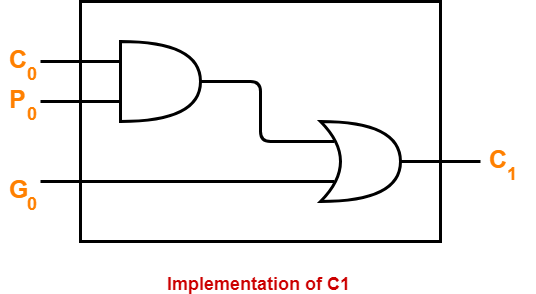### Implementation Of C2–

• The carry generator circuit for C2 is implemented as shown below.
• It requires 2 AND gates and 1 OR gate.

C2 = C0P0P1 + G0P1 + G1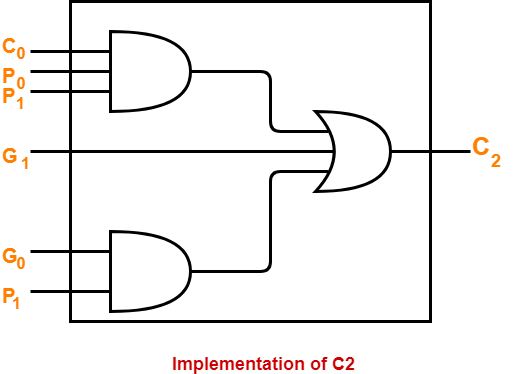### Implementation Of C3 & C4–

Similarly, we implement C3 and C4.

• Implementation of C3 uses 3 AND gates and 1 OR gate.
• Implementation of C4 uses 4 AND gates and 1 OR gate.

Total number of gates required to implement carry generators (provided carry propagators Pi and carry generators Gi) are-

• Total number of AND gates required for addition of 4-bit numbers = 1 + 2 + 3 + 4 = 10.
• Total number of OR gates required for addition of 4-bit numbers = 1 + 1 + 1 + 1 = 4.

### General Formula-

The following formula is used to calculate number of gates required for evaluating all carry bits-

 For a n-bit carry look ahead adder to evaluate all the carry bits, it requires-Number of AND gates = n(n+1) / 2Number of OR gates = n

• It generates the carry-in for each full adder simultaneously.
• It reduces the propagation delay.

• It involves complex hardware.
• It is costlier since it involves complex hardware.
• It gets more complicated as the number of bits increases.